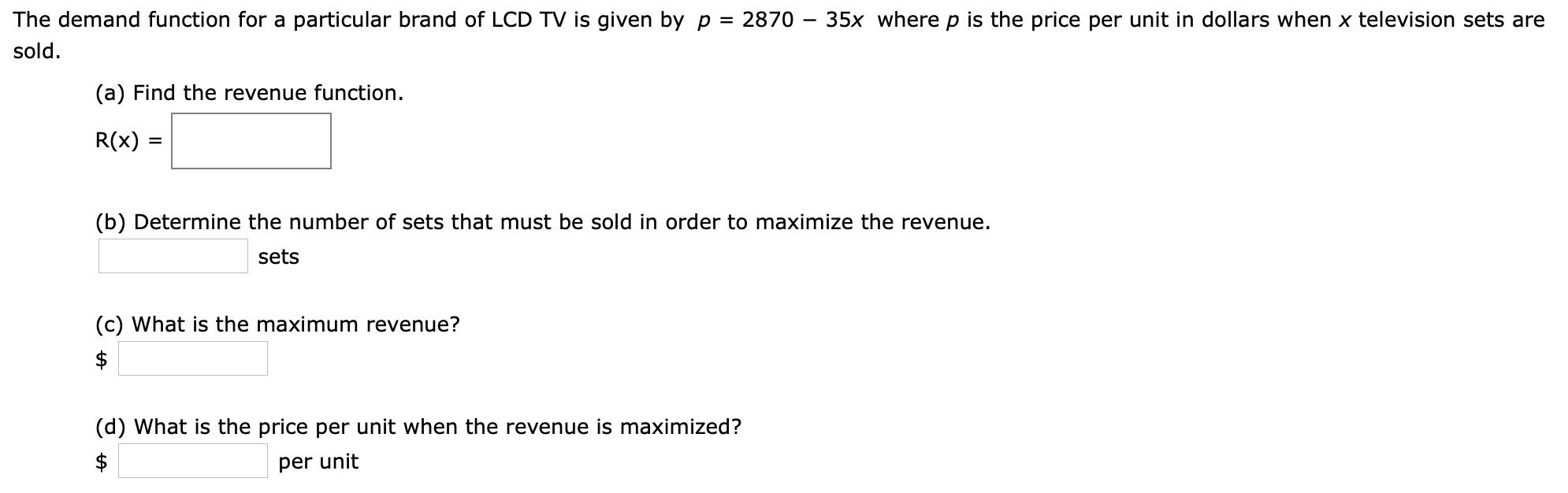# The demand function for a particular brand of LCD TV is given by p = 2870 - 35x where p is the price per unit in dollars when x television sets aresold(a) Find the revenue function.R(x)(b) Determine the number of sets that must be sold in order to maximize the revenuesets(c) What is the maximum revenue?\$(d) What is the price per unit when the revenue is maximized?\$per unit

Questionhelp_outlineImage TranscriptioncloseThe demand function for a particular brand of LCD TV is given by p = 2870 - 35x where p is the price per unit in dollars when x television sets are sold (a) Find the revenue function. R(x) (b) Determine the number of sets that must be sold in order to maximize the revenue sets (c) What is the maximum revenue? \$ (d) What is the price per unit when the revenue is maximized? \$ per unit fullscreen
check_circle

Step 1

As per norms, the first three questions are answered. To determine and find the critical points of the revenue function R(x) from the given data

Step 2

a) Apply the definition to get ...

### Want to see the full answer?

See Solution

#### Want to see this answer and more?

Solutions are written by subject experts who are available 24/7. Questions are typically answered within 1 hour.*

See Solution
*Response times may vary by subject and question.
Tagged in

### Math